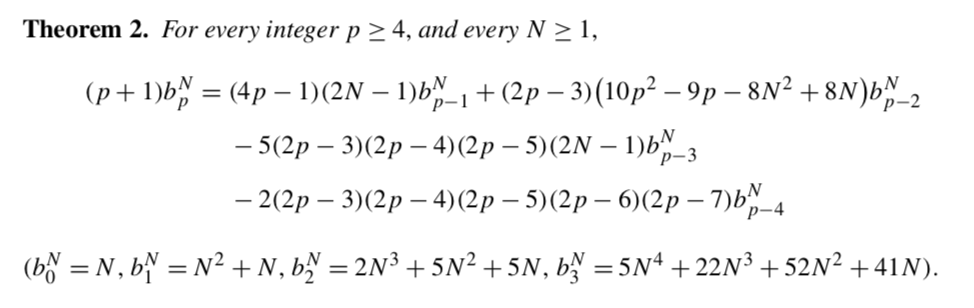# Gaussian integrals over the space of symmetric matrices

Let $S\in\mathcal S_N$ be a $N\times N$ symmetric matrix over the reals, and introduce the (normalised) gaussian measure $$\mathrm d\mu(S):=2^{-\frac 12N}\pi^{-\frac14N(N+1)}\exp\left[-\frac12\operatorname{tr}(S^2)\right]\mathrm dS$$ where $$\mathrm dS:=\prod_{i\le j\le N}\mathrm dS_{ij}$$

I am interested in the integral $$p_n(N):=\int_{\mathcal S_N}\operatorname{tr}(S^{2n})\mathrm d\mu(S)$$ which is in fact a polynomial in $N$ of degree $n+1$. It can be shown that the coefficients of these polynomials are given by a certain sum over all possible gluings of the $2n$-star (cf. Ref.1, exercise 3.2.12).

These polynomials can be computed explicitly (for example, with the aid of the Weyl integration formula, cf. this MO post, although I found it much more efficient to compute the integrals explicitly by means of Wick's theorem). The first few read \begin{equation} \begin{aligned} p_0(n) &= n\\ p_1(n) &= \frac12 n (1 + n)\\ p_2(n) &= \frac14 n (5 + 5 n + 2 n^2)\\ p_3(n) &= \frac18 n (41 + 52 n + 22 n^2 + 5 n^3)\\ p_4(n) &= \frac{1}{16} n (509 + 690 n + 374 n^2 + 93 n^3 + 14 n^4)\\ p_5(n) &= \frac{1}{32} n (8229 + 12143 n + 7150 n^2 + 2290 n^3 + 386 n^4 + 42 n^5)\\ p_6(n) &= \frac{1}{64} n (166377 + 258479 n + 167148 n^2 + 58760 n^3 + 12798 n^4 + 1586 n^5 + 132 n^6) \end{aligned} \end{equation}

My question: is there any known formula to compute these polynomials efficiently (say, in the form of an explicit recurrence relation, or a closed-form generating function, etc.)?

Note that $p_n(1)=(2n-1)!!$, which is not too difficult to prove.

If we integrate over hermitian matrices instead of symmetric matrices, the answer to the question above is affirmative (the recurrence relation and the generating functional can be found in Ref.1 §1). In the symmetric case, the best I could find was Ref.2, which is not nearly as explicit as I would like (if the recurrence relation or the explicit generating function for $p_n(N)$ is somewhere there, I couldn't find it myself).

As per Wick's theorem, I am lead to believe it should be useful to express the polynomials $p_n(N)$ using as a basis the bionomial coefficients instead of the powers $\{1,N,N^2,\dots\}$: $$p_n(N):=\sum_{j=1}^{n+1}\frac{1}{2^n}\alpha_{n,j}\binom{N}{j}$$ where the coefficients can be arranged in a Pascal-like triangle: \begin{equation} \begin{aligned} &\hspace{127pt}1\\ &\hspace{100pt}1\hspace{50pt} 1\\ &\hspace{80pt}6\hspace{40pt} 11\hspace{40pt} 6\\ &\hspace{60pt}60\quad\qquad 153\quad\qquad 156\quad\qquad 60\\ &\qquad\qquad840\qquad 2673\qquad 3846\qquad 2796\qquad 840\\ &\qquad15120\quad 56715\quad 102960\quad 106560\quad 60960\quad 15120\\ &332640\ 1420695\ 3066390\ 4032360\ 3304080\ 1568880\ 332640\\ \end{aligned} \end{equation}

I couldn't find a recurrence relation for the $\alpha_{n,j}$ coefficients either, so I'm not sure this representation is actually more useful than the previous one, but I wanted to include it as well just in case. Note that the triangle is almost symmetric, so that may mean something.

Note: OEIS was unable to recognise any of these sequences/polynomials. Could it be useful to submit them?

References.

1. Sergei K. Lando, Alexander K. Zvonkin - Graphs on Surfaces and Their Applications (Springer, 2004).

2. I.P. Goulden, D.M. Jackson, Maps in locally orientable surfaces, and integrals over real symmetric matrices, Canadian J. Math. 49, 1997, 865-882.

• I wasn't sure which tags to use. Feel free to fix them. – AccidentalFourierTransform Feb 6 '18 at 16:26
• This is purely recreational. I am a physicists so my mathematical background is not strong. More so in the case of these kinds of problems, in which I am a complete beginner but I found to be quite fun. Feel free to suggest references that I might find interesting. Cheers! – AccidentalFourierTransform Feb 6 '18 at 16:33
• The polynomials appear to satisfy an almost Appell sequence: $$p'_n(N)=\left(2n-1+\frac{2^{j-2} j!}{(2 j-3)!!}\frac{1}{N}+\cdots\right)p_{n-1}(N)$$ Perhaps they form a Sheffer sequence? – AccidentalFourierTransform Feb 6 '18 at 17:11

The desired recursion formula for the moment $b_p^N\equiv E\,[\,{\rm tr}\,(S_N^{2p})]$ isI notice a difference in normalization, you'll want to divide $b_p^N$ by $2^p$.